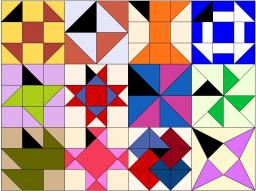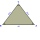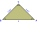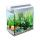# Isosceles triangle

Calculate the perimeter of isosceles triangle with arm length 73 cm and base length of 48 cm.

p =  194 cm

### Step-by-step explanation:Did you find an error or inaccuracy? Feel free to write us. Thank you!Tips to related online calculators
Do you want to convert length units?

#### You need to know the following knowledge to solve this word math problem:

We encourage you to watch this tutorial video on this math problem:

## Related math problems and questions:

• TrianglesEquilateral triangle with side 40 cm has the same perimeter as an isosceles triangle with arm of 45 cm. Calculate the base x of an isosceles triangle.
• Arm-legCalculate the length of the base of an isosceles triangle with a circumference 224 cm if the arm length is 68 cm.
• Base of an isosceles triangleCalculate the size of the base of an isosceles triangle, the height is 5 cm and the length of the arm is 6.5 cm. What is the perimeter of this triangle?
• Arm and baseThe isosceles triangle has a circumference of 46 cm. Calculate its area if the arm is 5 cm longer than the base.
• ArmCalculate the length of the arm r of isosceles triangle ABC, with base |AB| = 14 cm and a height v=18 cm.
• Isosceles triangleCalculate the size of the interior angles and the length of the base of the isosceles triangle if the length of the arm is 17 cm and the height to the base is 12 cm.
• Isosceles triangleThe perimeter of an isosceles triangle is 112 cm. The length of the arm to the length of the base is at ratio 5:6. Find the triangle area.
• Isosceles triangleThe circumference of the isosceles triangle is 32.5 dm. Base length is 153 cm. How long is the leg of this triangle?
• Isosceles + prismCalculate the volume of the perpendicular prism if its height is 17.5 cm and the base is an isosceles triangle with a base length of 5.8 cm and an arm length of 3.7 cm
• Isosceles triangleIn an isosceles triangle, the arm's length and the length of the base are in ratio 3 to 5. What is the length of the arm?
• ISO Triangle V2The perimeter of RR triangle (isosceles) is 474 m, and the base is 48 m longer than the arms. Calculate the area of this triangle.
• A isoscelesA isosceles triangle has an area of 168 cm2 and it's added height and base is 370 cm. What are the measurements of it's height and base?
• AquariumAquarium is rectangular box with square base containing 76 liters of water. Length of base edge is 42 cm. To what height the water level goes?
• Aquarium IICalculate how much glass we need to build an aquarium with a rectangular shape with base 70 cm × 70 cm and a height of 70 cm, if the waste is 2%. Aquarium haven't top glass.
• BaseCompute base of an isosceles triangle, with the arm a=20 cm and a height above the base h=10 cm.
• Medians of isosceles triangleThe isosceles triangle has a base ABC |AB| = 16 cm and 10 cm long arm. What is the length of medians?
• Isosceles trapezoidFind the area of an isosceles trapezoid with bases of 8cm and 72mm. The height of the trapezoid is equal to three-quarters of the longer base.